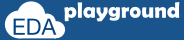# Solve Before Constraints

Solve before is the constraint property. solve before is used inside the constraint block to specify the order of constraint solving. If the variables are dependent, due to the bidirectional nature of constraints value of one variable will influence the value of another variable.

• solve before constraints are used to force the constraint solver to choose the order in which constraints are solved.
• constraint solver will give equal weight-age to all the possible values. i.e On multiple randomization solver should assign all the possible values.

```class pakcet;
rand bit       a;
rand bit [3:0] b;

constraint a_b { (a == 1) -> b == 0; }
endclass
```

Possible value of,
```a -> 0 and 1;  // 2 possible values
b -> 0 to  15; // 16 possible values```

As 'b' value ranges 0:15, Probability of getting b == 0 is very less.
so it will impact the value of a,  i.e if value of b != 0, which will lead to value of 'a' to become '0'.

What if in some situations it is required to generate a value of 'a' to '1' more frequently. by using 'solve before' it can be done.

Writing below constraint will direct solver to solve 'a' first, so more frequently a will take value of 1.

```constraint sab { solve a before b;}
```

## Solve before constraint example

### without solve before

The below example is the example explained above,
this example is without solve before constraint, so we can see the simulation result that the occurrence of value a == 1 is less.

```class packet;
rand bit       a;
rand bit [3:0] b;

constraint a_b { (a == 1) -> b == 0; }
endclass

module inline_constr;
initial begin
packet pkt;
pkt = new();
repeat(10) begin
pkt.randomize();
\$display("\tValue of a = %0d, b = %0d",pkt.a,pkt.b);
end
end
endmodule
```

Simulator Output

Value of a = 0, b = 6
Value of a = 0, b = 3
Value of a = 1, b = 0
Value of a = 0, b = 15
Value of a = 0, b = 7
Value of a = 0, b = 2
Value of a = 0, b = 15
Value of a = 0, b = 4
Value of a = 0, b = 7
Value of a = 0, b = 11
Execute the above code on### with solve before

this example is to solve before constraint, so we can see the simulation result that the occurrence of value a == 1 is more than without solve before constraint.

```class packet;
rand bit       a;
rand bit [3:0] b;

constraint sab { solve a before b;}
constraint a_b { (a == 1) -> b == 0;}
endclass

module inline_constr;
initial begin
packet pkt;
pkt = new();
repeat(10) begin
pkt.randomize();
\$display("\tValue of a = %0d, b = %0d",pkt.a,pkt.b);
end
end
endmodule
```

Simulator Output

Value of a = 0, b = 9
Value of a = 0, b = 14
Value of a = 0, b = 3
Value of a = 0, b = 13
Value of a = 1, b = 0
Value of a = 1, b = 0
Value of a = 1, b = 0
Value of a = 0, b = 5
Value of a = 0, b = 3
Value of a = 0, b = 4

Execute the above code on